Test: Reasoning Ability - 5

# Test: Reasoning Ability - 5 - Banking Exams

Test Description

## 20 Questions MCQ Test IBPS PO Mock Test Series & Past Year Papers - Test: Reasoning Ability - 5

Test: Reasoning Ability - 5 for Banking Exams 2023 is part of IBPS PO Mock Test Series & Past Year Papers preparation. The Test: Reasoning Ability - 5 questions and answers have been prepared according to the Banking Exams exam syllabus.The Test: Reasoning Ability - 5 MCQs are made for Banking Exams 2023 Exam. Find important definitions, questions, notes, meanings, examples, exercises, MCQs and online tests for Test: Reasoning Ability - 5 below.
Solutions of Test: Reasoning Ability - 5 questions in English are available as part of our IBPS PO Mock Test Series & Past Year Papers for Banking Exams & Test: Reasoning Ability - 5 solutions in Hindi for IBPS PO Mock Test Series & Past Year Papers course. Download more important topics, notes, lectures and mock test series for Banking Exams Exam by signing up for free. Attempt Test: Reasoning Ability - 5 | 20 questions in 10 minutes | Mock test for Banking Exams preparation | Free important questions MCQ to study IBPS PO Mock Test Series & Past Year Papers for Banking Exams Exam | Download free PDF with solutions
 1 Crore+ students have signed up on EduRev. Have you?
Test: Reasoning Ability - 5 - Question 1

### Directions: Read both the conclusions and then decide which of the given conclusions logically follows from the given statements disregarding commonly known facts. Give Answer: (a) If Only conclusion I follows. (b) If Only conclusion II follows. (c) If either conclusion I or II follows. (d) If Neither conclusion I nor II follows. (e) If both conclusions I and II follow. Statement Only a few Cakes are Lake All Lake are Bake No Bake is Fake Conclusion I. All Cake can be Fake II. Some Bake is not a Cake

Detailed Solution for Test: Reasoning Ability - 5 - Question 1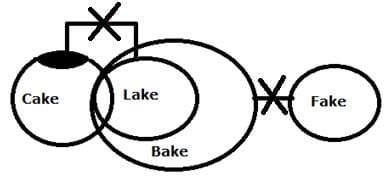Neither conclusion I nor II follows

Test: Reasoning Ability - 5 - Question 2

### Directions: Read both the conclusions and then decide which of the given conclusions logically follows from the given statements disregarding commonly known facts. Give Answer: (a) If Only conclusion I follows. (b) If Only conclusion II follows. (c) If either conclusion I or II follows. (d) If Neither conclusion I nor II follows. (e) If both conclusions I and II follow. Statement Only a few Mail are Tail All Mail are Rail No Rail is Nail Conclusion I. No Mail is Nail II. Some Rail is not a Tail

Detailed Solution for Test: Reasoning Ability - 5 - Question 2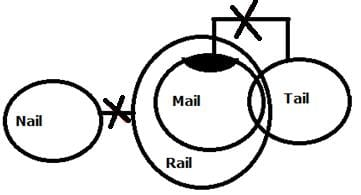Both conclusions I and II follow.

Test: Reasoning Ability - 5 - Question 3

### Directions: In each of the following questions, the relationship between different elements is shown in the statements followed by some conclusions. Find the conclusion which is definitely true. Statements: Q > T > V = I; L > B > R ≥ I; X ≤ Y ≤ L Conclusions:

Detailed Solution for Test: Reasoning Ability - 5 - Question 3

(a) Q > B (B > R ≥ I = V < T < Q)→False

(b) I > Y (Y ≤ L > B > R ≥ I)→False

(c) L > V (L > B > R ≥ I = V)→True

(d) X ≤ B (X ≤ Y ≤ L >B) →False

(e) R > Q (R ≥ I = V < T < Q) →False

Test: Reasoning Ability - 5 - Question 4

Directions: In each of the following questions, the relationship between different elements is shown in the statements followed by some conclusions. Find the conclusion which is definitely true.

Statements:

K < B > S = I;
H = N ≥ R > X;
H ≤ L = I

Conclusions:

Detailed Solution for Test: Reasoning Ability - 5 - Question 4

(a) K > H (K < B > S = I = L ≥ H) →False

(b) R ≤ S (S = I = L ≥ H = N ≥ R)→True

(c) R < I (I = L ≥ H = N ≥ R)→False

(d) L ≥ X (L ≥ H = N ≥ R > X)→False

(e) K < L (K < B > S = I = L)→False

Test: Reasoning Ability - 5 - Question 5

Directons: Read both the conclusions and then decide which of the given conclusions logically follows from the given statements disregarding commonly known facts.

(a) If Only conclusion I follows.

(b) If Only conclusion II follows.

(c) If either conclusion I or II follows.

(d) If Neither conclusion I nor II follows.

(e) If both conclusions I and II follow.

Statement

Only a few Back are Lack

No Lack is Rack

All Racks are Sack

Conclusion

I. All Back can never be Rack

II. Some Lack being Sack is a possibility

Detailed Solution for Test: Reasoning Ability - 5 - Question 5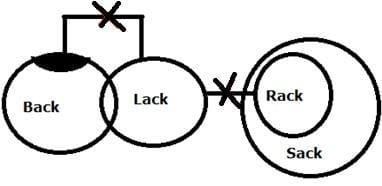Both conclusions I and II follow.

Test: Reasoning Ability - 5 - Question 6

Directions: Study the following information carefully and answer the below questions.

In a certain code language

‘ Account Receivable Cash Flow ’ means ‘ ah ib sh fm’,

‘ Cash Debit Account Payable’ means ‘ sh dt ah yh’,

Cash Credit Payable Capital’ means ‘ sh ef pt yh’,

Balance Receivable Debit Bond’ means ‘ ln nd dt ib ’

Q. What does "ib" represent in a code language?

Detailed Solution for Test: Reasoning Ability - 5 - Question 6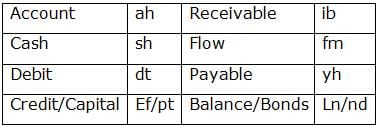Test: Reasoning Ability - 5 - Question 7

Directions: Study the following information carefully and answer the below questions.

In a certain code language

‘ Account Receivable Cash Flow ’ means ‘ ah ib sh fm’,

‘ Cash Debit Account Payable’ means ‘ sh dt ah yh’,

Cash Credit Payable Capital’ means ‘ sh ef pt yh’,

Balance Receivable Debit Bond’ means ‘ ln nd dt ib ’

Q. If “Balance flow” is coded as “ln fm”, then what does “nd sh ah” represent in the code language?

Detailed Solution for Test: Reasoning Ability - 5 - Question 7Test: Reasoning Ability - 5 - Question 8

Directions: Study the following information carefully and answer the below questions.

In a certain code language

‘ Account Receivable Cash Flow ’ means ‘ ah ib sh fm’,

‘ Cash Debit Account Payable’ means ‘ sh dt ah yh’,

Cash Credit Payable Capital’ means ‘ sh ef pt yh’,

Balance Receivable Debit Bond’ means ‘ ln nd dt ib ’

Q. What is the code for ‘Cash Payable’ in the given language?

Detailed Solution for Test: Reasoning Ability - 5 - Question 8Test: Reasoning Ability - 5 - Question 9

Directions: Study the following information carefully and answer the below questions.

In a certain code language

‘ Account Receivable Cash Flow ’ means ‘ ah ib sh fm’,

‘ Cash Debit Account Payable’ means ‘ sh dt ah yh’,

Cash Credit Payable Capital’ means ‘ sh ef pt yh’,

Balance Receivable Debit Bond’ means ‘ ln nd dt ib ’

Q. If “Credit Receivable” is coded as “ef ib”, What is the code for ‘Capital flow’ in the given language?

Detailed Solution for Test: Reasoning Ability - 5 - Question 9Test: Reasoning Ability - 5 - Question 10

How many such pairs of letters are in the word “ CHRISTMAS” which has as many letters between them in the English alphabetical order(from both forward and backward direction)?

Detailed Solution for Test: Reasoning Ability - 5 - Question 10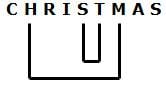Test: Reasoning Ability - 5 - Question 11

Directions: Study the following information carefully and answer the below questions

G is the father-in-law of T who is the only daughter of Q. S is the father of F who is the nephew of B. C is the brother-in-law of S. L is the daughter of T who is the daughter-in-law of M. B is the only daughter of M. B is an unmarried person in the family.

Q. Who among the following person is the spouse of S?

Detailed Solution for Test: Reasoning Ability - 5 - Question 11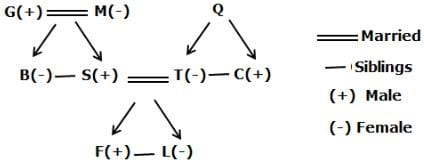Test: Reasoning Ability - 5 - Question 12

Directions: Study the following information carefully and answer the below questions

G is the father-in-law of T who is the only daughter of Q. S is the father of F who is the nephew of B. C is the brother-in-law of S. L is the daughter of T who is the daughter-in-law of M. B is the only daughter of M. B is an unmarried person in the family.

Q. How C is related to T?

Detailed Solution for Test: Reasoning Ability - 5 - Question 12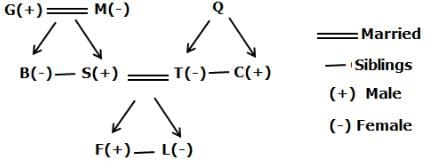Test: Reasoning Ability - 5 - Question 13

Directions: Study the following information carefully and answer the below questions

Mr Jina starts walking from point X towards the north for 9m to reach point F where he turns to the west direction and walks for 10m to reach point G where he turns to the left and walks for 4m to reach point T. Then he turns to the left and walks for 7m to reach point B where he turns to the south and walks for 5m to reach Point S. Then he turns to the right and walks for 12m to reach point L where he turns to the left and walks for 11m to reach point Q.

Q. From point Q, If Jina walks for 15m to the east and turns to the north, then which of the following point she will reach first?

Detailed Solution for Test: Reasoning Ability - 5 - Question 13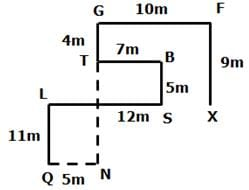Test: Reasoning Ability - 5 - Question 14

Directions: Study the following information carefully and answer the below questions

Mr Jina starts walking from point X towards the north for 9m to reach point F where he turns to the west direction and walks for 10m to reach point G where he turns to the left and walks for 4m to reach point T. Then he turns to the left and walks for 7m to reach point B where he turns to the south and walks for 5m to reach Point S. Then he turns to the right and walks for 12m to reach point L where he turns to the left and walks for 11m to reach point Q.

Q. If point N is east of Point Q and south of point T, then what is the distance between T and N?

Detailed Solution for Test: Reasoning Ability - 5 - Question 14Test: Reasoning Ability - 5 - Question 15

Directions: Study the following information carefully and answer the below questions

Mr Jina starts walking from point X towards the north for 9m to reach point F where he turns to the west direction and walks for 10m to reach point G where he turns to the left and walks for 4m to reach point T. Then he turns to the left and walks for 7m to reach point B where he turns to the south and walks for 5m to reach Point S. Then he turns to the right and walks for 12m to reach point L where he turns to the left and walks for 11m to reach point Q.

Q. What is the direction of point S with respect to point X?

Detailed Solution for Test: Reasoning Ability - 5 - Question 15Test: Reasoning Ability - 5 - Question 16

Directions: Study the following information carefully and answer the below questions.

Nine persons- A, B, C, D, E, F, G, H and I are born in different years- 1965, 1968, 1973, 1981, 1983, 1989, 1993, 1996, and 2000 but not necessarily in the same order. Their ages are calculated with respect to the year 2021.

Only two persons are born between D and H who is four years elder than B. The number of persons born between D and B is the same as the number of persons born between H and G. The sum of the ages of H and G is more than 70. The sum of the ages of F and I is 101. The number of persons elder than I is the same as the number of persons younger than E. C is elder than A.

Q. How many persons are born between I and A?

Detailed Solution for Test: Reasoning Ability - 5 - Question 16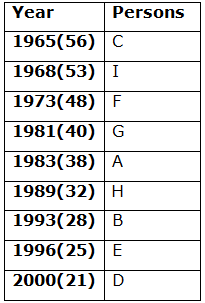We have,

• Only two persons are born between D and H who is four years elder than B.

From the above condition, there are three possibilities.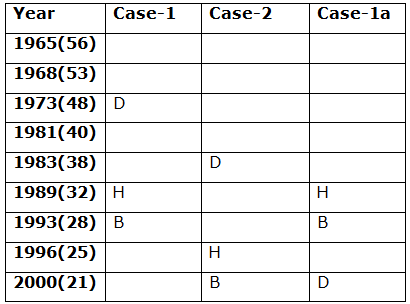Again we have,

• The number of persons born between D and B is the same as the number of persons born between H and G.
• The sum of the ages of H and G is more than 70.

From the above condition, case-2 gets eliminated.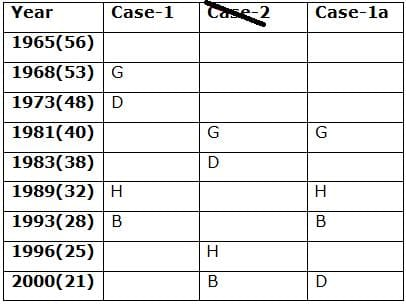Again we have,

• The sum of the ages of F and I is 101.
• The number of persons elder than I is the same as the number of persons younger than E.
• C is elder than A.

From the above condition, case-1 gets eliminated. Case-1a shows the final arrangement.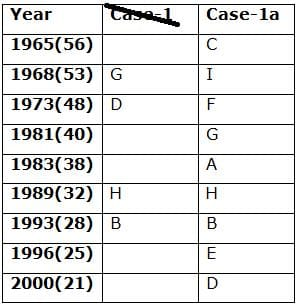Test: Reasoning Ability - 5 - Question 17

Directions: Study the following information carefully and answer the below questions.

Nine persons- A, B, C, D, E, F, G, H and I are born in different years- 1965, 1968, 1973, 1981, 1983, 1989, 1993, 1996, and 2000 but not necessarily in the same order. Their ages are calculated with respect to the year 2021.

Only two persons are born between D and H who is four years elder than B. The number of persons born between D and B is the same as the number of persons born between H and G. The sum of the ages of H and G is more than 70. The sum of the ages of F and I is 101. The number of persons elder than I is the same as the number of persons younger than E. C is elder than A.

Q. In which of the following year does E was born?

Detailed Solution for Test: Reasoning Ability - 5 - Question 17We have,

• Only two persons are born between D and H who is four years elder than B.

From the above condition, there are three possibilities.Again we have,

• The number of persons born between D and B is the same as the number of persons born between H and G.
• The sum of the ages of H and G is more than 70.

From the above condition, case-2 gets eliminated.Again we have,

• The sum of the ages of F and I is 101.
• The number of persons elder than I is the same as the number of persons younger than E.
• C is elder than A.

From the above condition, case-1 gets eliminated. Case-1a shows the final arrangement.Test: Reasoning Ability - 5 - Question 18

Directions: Study the following information carefully and answer the below questions.

Nine persons- A, B, C, D, E, F, G, H and I are born in different years- 1965, 1968, 1973, 1981, 1983, 1989, 1993, 1996, and 2000 but not necessarily in the same order. Their ages are calculated with respect to the year 2021.

Only two persons are born between D and H who is four years elder than B. The number of persons born between D and B is the same as the number of persons born between H and G. The sum of the ages of H and G is more than 70. The sum of the ages of F and I is 101. The number of persons elder than I is the same as the number of persons younger than E. C is elder than A.

Q. Four of the five among the following are similar in such a way to form a group, who among the following doesn’t belong to the group?

Detailed Solution for Test: Reasoning Ability - 5 - Question 18We have,

• Only two persons are born between D and H who is four years elder than B.

From the above condition, there are three possibilities.Again we have,

• The number of persons born between D and B is the same as the number of persons born between H and G.
• The sum of the ages of H and G is more than 70.

From the above condition, case-2 gets eliminated.Again we have,

• The sum of the ages of F and I is 101.
• The number of persons elder than I is the same as the number of persons younger than E.
• C is elder than A.

From the above condition, case-1 gets eliminated. Case-1a shows the final arrangement.Test: Reasoning Ability - 5 - Question 19

Directions: Study the following information carefully and answer the below questions.

Nine persons- A, B, C, D, E, F, G, H and I are born in different years- 1965, 1968, 1973, 1981, 1983, 1989, 1993, 1996, and 2000 but not necessarily in the same order. Their ages are calculated with respect to the year 2021.

Only two persons are born between D and H who is four years elder than B. The number of persons born between D and B is the same as the number of persons born between H and G. The sum of the ages of H and G is more than 70. The sum of the ages of F and I is 101. The number of persons elder than I is the same as the number of persons younger than E. C is elder than A.

Q. What is the sum of the ages of B and I?

Detailed Solution for Test: Reasoning Ability - 5 - Question 19We have,

• Only two persons are born between D and H who is four years elder than B.

From the above condition, there are three possibilities.Again we have,

• The number of persons born between D and B is the same as the number of persons born between H and G.
• The sum of the ages of H and G is more than 70.

From the above condition, case-2 gets eliminated.Again we have,

• The sum of the ages of F and I is 101.
• The number of persons elder than I is the same as the number of persons younger than E.
• C is elder than A.

From the above condition, case-1 gets eliminated. Case-1a shows the final arrangement.Test: Reasoning Ability - 5 - Question 20

Directions: Study the following information carefully and answer the below questions.

Nine persons- A, B, C, D, E, F, G, H and I are born in different years- 1965, 1968, 1973, 1981, 1983, 1989, 1993, 1996, and 2000 but not necessarily in the same order. Their ages are calculated with respect to the year 2021.

Only two persons are born between D and H who is four years elder than B. The number of persons born between D and B is the same as the number of persons born between H and G. The sum of the ages of H and G is more than 70. The sum of the ages of F and I is 101. The number of persons elder than I is the same as the number of persons younger than E. C is elder than A.

Q. If A’s brother was born two years after A, then what is the age of A’s brother?

Detailed Solution for Test: Reasoning Ability - 5 - Question 20We have,

• Only two persons are born between D and H who is four years elder than B.

From the above condition, there are three possibilities.Again we have,

• The number of persons born between D and B is the same as the number of persons born between H and G.
• The sum of the ages of H and G is more than 70.

From the above condition, case-2 gets eliminated.Again we have,

• The sum of the ages of F and I is 101.
• The number of persons elder than I is the same as the number of persons younger than E.
• C is elder than A.

From the above condition, case-1 gets eliminated. Case-1a shows the final arrangement.## IBPS PO Mock Test Series & Past Year Papers

33 docs|72 tests
Information about Test: Reasoning Ability - 5 Page
In this test you can find the Exam questions for Test: Reasoning Ability - 5 solved & explained in the simplest way possible. Besides giving Questions and answers for Test: Reasoning Ability - 5, EduRev gives you an ample number of Online tests for practice

33 docs|72 tests